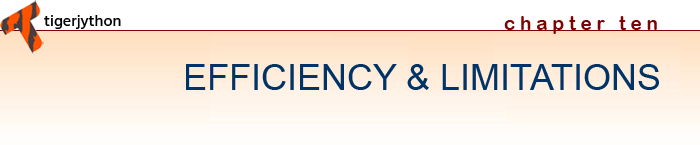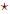Learning ObjectivesYou can illustrate with some examples that there are problems that can be formulated algorithmically but which cannot be solved with the computer.You know what is meant by the polynomial and non-polynomial time complexity of a program.You can explain the halting problem based on the example of the 3n+1 algorithm.You know what is meant by combinatorial explosion.You can search a graph using backtracking.You know some classic encryption methods and can implement them in a program.You know the concept of the finite state machine and know how to implement a simple finite-state machine..